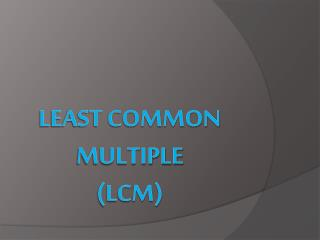# Least Common Multiple (LCM) - PowerPoint PPT PresentationDownload PresentationLeast Common Multiple (LCM)

Least Common Multiple (LCM)Download Presentation## Least Common Multiple (LCM)

- - - - - - - - - - - - - - - - - - - - - - - - - - - E N D - - - - - - - - - - - - - - - - - - - - - - - - - - -
##### Presentation Transcript

1. Least Common Multiple(LCM)

2. Definition The smallest postive number that is a multiple of two or more given numbers.

3. Method #1: Make a List STEP 1: List the first 10 multiples of the greater number. STEP 2: List the multiples of the lesser number. Stop when you get to a multiple that was in your first list. NOTE: You may find that after listing several multiples for the lesser number that you still do not have a common multiple. If this is the case, list the next 10 multiples of the greater number and then continue your list for the lesser number.

4. Example #1 Find the least common multiple of 6 and 9 Multiples of 9: _____________________________ Multiples of 6: _____________________________ Least Common Multiple of 6 and 9: __ 9, 18, 27, 36, 45, 54, 63, 72, 81, 90 6, 12, 18 18

5. Example #2 Find the least common multiple of 5, 8, and 10. Multiples of 10: ____________________________ Multiples of 8: ____________________________ Least Common Multiple of 5, 8, and 10: __ 10, 20, 30, 40, 50, 60, 70, 80, 90, 100 8, 16, 24, 32, 40 Since you have a common multiple for 10 and 8, ask yourself, “is that number is also a multiple of 5?” If it is you have the LCM, if not continue to the next common multiple of 10 and 8. 40

6. Method #2: Upside Down Cake ***This will be very similar to how we found the GCF*** but there is an added twist at the end Example 1: Find the LCM(10,12) Find the GCF of the numbers given 10 12 2 5 6 Multiply each of the numbers circled to find the LCM. 2 • 5 • 6 = 60  the LCM of 10 and 12 is 60

7. Method #2: Upside Down Cake Example 2: Find the LCM(15,25) 15 25 5 Find the GCF of the numbers given 3 5 Multiply each of the numbers circled to find the LCM. 5 • 3 • 5 = 75  the LCM of 15 and 25 is 75

8. Method #2: Upside Down Cake Example 3: Find the LCM(6,8,12) 6 8 12 2 Find the GCF of the numbers given 2 3 4 6 Since two of the numbers share a factor… continue Since two of the numbers share a factor… continue 3 3 2 3 1 2 1 Multiply each of the numbers circled to find the LCM. 2 • 2 • 3 • 1 • 2 • 1 = 24  the LCM of 6, 8, and 12 is 24

9. PAY ATTENTION!!!! IF THE NUMBERS HAVE A gcF OF 1, THEN THE LCM IS THE PRODUCT OF ALL THE NUMBERS MULTIPLIED TOGETHER!!!! Example: LCM(2,5,13): The GCF of these numbers is 1 2 • 5 • 13 = 130

10. Rod helped his mom plant a vegetable garden. Rod planted a row every 30 minutes, and his mom planted a row every 20 minutes. If they started together, how long will it be before they both finish a row at the same time? Least Common Multiple of 30 and 20: ________ 60 minutes

11. Three bike riders ride around a circular path. The first rider circles the path in 4 minutes, the second in 9 minutes, and the third in 12 minutes. If they all start at the same place at the same time, and go in the same direction, after how many minutes will they meet at the starting point? Least Common Multiple of 4, 9, and 12: ________ 36 minutes